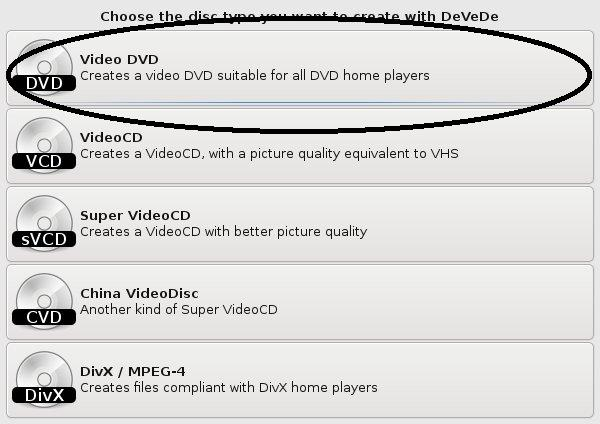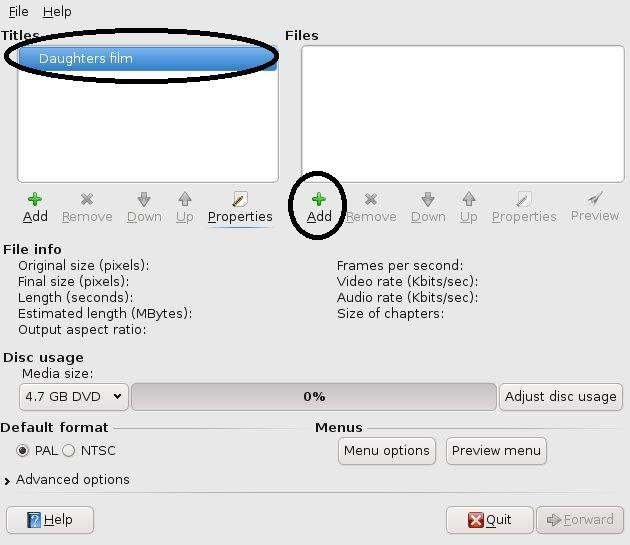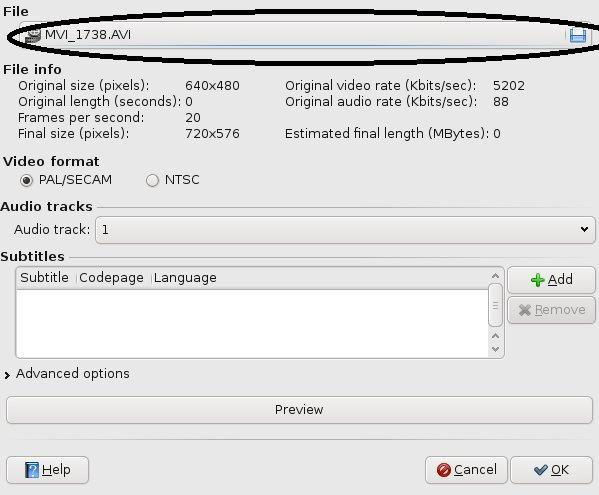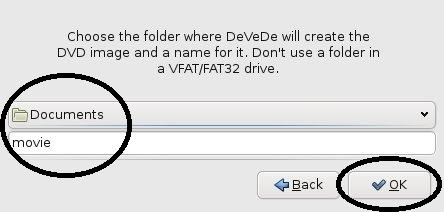## DeVeDe – convert video files to DVD’s

To convert video files into Video DVD’s for general usage well there is allot of applications out there that can do the job, command line (bash) if you want to or just use a GUI. I personally used to use a commmand line but now I am using a GUI because personally it just is as easy and sometimes it is nice to use a gui and not have to think as such.

The application that I use is called DeVeDe and it just makes the conversion very nice. Here is a step by step guide to use it.

Here is the output types that you can choose from, I have never used anything else but Video DVD.Start the create of the DVD structure, e.g. files and titles names.Properties of the title name(s) that you would like to come up on the main menu of the DVD.Add the files that you want to associated with the title on the main menu of the DVD, these could be of any type of file Xvid, MPEG, etc.Titles and the files that are associated with the DVD structure.Create the DVD, this will progress to where to place the ISO image of the DVD structure.Create the Full DVD StructureFinished!! all done.

## Virtual Box

After looking over the virtualization with different setups, KVM and VirtualBox I decided to use the virtualbox method because it just appeared to be nicer and with a nice GUI per machine just felt nice.

With the additions of the Guest Additions to allow for sharing of folders from the host machine to the virtual one and also the mouse was no longer taken within the virtual machine, I just liked it and to setup a bridge for the virtual machine to use for the networking I created a small script that does the job of deleting the KVM inserting modules into K/Ubuntu.

Here it is

```#!/bin/sh   # to remove any kernel modules for kvm and then bring up the bridge for eth0 TMP=`lsmod | grep kvm` RESULT=`echo \$TMP | cut -d \ -f 1,4`   if [ -n "\$RESULT" ]; then   for i in 1 2 do rmmod `echo \$RESULT | cut -d \ -f \$i` done else echo "No kernel modules" fi   # setup the eth0 for working with the bridge ifconfig eth0 0.0.0.0 #ifconfig br0 down   #setup the bridge and bring it up brctl addbr br0 brctl addif br0 eth0   ifconfig br0 up```

## Constant casting

Constant casting is when you are for example calling a external library function that does not understand the const (constant) type and so you will need to take it off because the program will do something funny otherwise, crash. etc..

Here is a example code of a const_cast

```#include <iostream>   using namespace std;   int exampleextenalfuction(int value) { // you can alter the value if you want to, since it is not longer a constant value return value + value; }   int main() { const int constvalue = 2;   cout << constvalue << endl;   cout << "Calling a external function that does not use constant types" << endl;   cout << exampleextenalfuction(const_cast<int&>(constvalue)) << endl; }```

## Static casting

Static casting is when you want to convert one type into another, static casting is used allot of the time without even knowing. For example

```int value = 2; float t = float(value);```

the float(value) is a static casting of converting one value into another. Below is a full code example

```#include <iostream>   using namespace std; int main() { int i = 3; // the float(<value)> is basically a static cast from a interger value into a float cout << float(i) << endl; cout << static_cast<float>(i) << endl; }```

It is very small since static_cast’ing is very basic in nature, but the only problem is that you have to check to make sure that there is a error e.g not NULL.

## Execution order

Execution order of programs code is very much a vital thing to understand and where some fault finds will take a long while to figure out. Basically like in maths where there is a order of calculating, well in coding structures and also multitasking and multi-threading setups the execution order may be incorrect for lines of code.

Here is some examples, the first is when will a function be called and the later when post/pre incrementation will take place.

```#include <iostream>   using namespace std;   int value =1;   int setvalue2() { cout << "setting value"<<endl; value = 2; return value; }   int returnvalue() { cout << "renting value"<< endl; return value; }   int main() { // depending on the order of execution the value may be /* setvalue2 called first (setvalue2 = 2 / returnvalue = 2) = 1 returnvalue called first (setvalue2 = 2 / returnvalue = 1) = 2 */ cout << setvalue2() / returnvalue() << endl;   int i; i = i++ - ++i; // not sure what i will be because the pre/post increaments i = 3, i++, i++; // i will equal 5 because in correct order. }```

## Dynamic Casting

Dynamic casting, means that you can convert one object into another that is off the same type. For example, if you had a base class called Shape and a inherited class called Rectangle then you are able to convert a Shape object into a Rectangle.

`Rectangle *rec = dynamic_cast<Shape *>(shapeobject);`

sort of thing, there has to be a virtual function within the base class otherwise the compiler will complain, but apart from that that is about it.

Dynamic casting allows for NULL returns which is the best thing, because you can test to see if the casting actually worked and not to do anything silly on a NULL object which would crash the program.

Pointer casting uses the sytnax

`<type> *p_subclass = dynamic_cast<<type> *>( p_obj );`

Reference will not throw an error/expcetion so will need to check std::bad_cast< typeinfo header >, here is the syntax

`<type> subclass = dynamic_cast<<type> &>( ref_obj );`

Hopefully this will make more sense for how and why it works.

```#include <iostream>   using namespace std;   class classA { public : int x; // should be private char y; // should be private   classA();   virtual ~classA() {}; // need to have a virtual function for dynamic casting };   // basic classA constructor classA::classA() { cout << "classA" << endl; x = 1; y = 'a'; }     class classB : public classA { public : int b; // should be private   classB();   ~classB() {}; // complete the virtual };   // basic classB constructor classB::classB() { cout << "classB" << endl; x = 2; y = 'b'; }   int main() { classA newa; // classA obj cout << "class A constructed" << endl; classB newb; // classB obj cout << "class B constructed" << endl;   cout << "NewA X " << newa.x << endl; cout << "NewB X " << newb.x << endl;   // point a classB to a already created classB object classB *normalB = &newb; // dynamic_cast a normalB object (newb) to another classA object classA *dynA = dynamic_cast<classB *>(normalB); cout << "dynamic A X " << dynA->x << endl;   // does not work, because you cannot convert classA into a classB, but if classA was pointing to a classB type. classA *normalA = &newa; classB *dynB = dynamic_cast<classB *>(normalA); if (dynB) // above produces a 0 because invalid. { cout << "dynamic B X " << dynB->x << endl; cout << "dynamic B X " << dynB->b << endl; }   // this does work because it is converting from a pointer of classB type, which was "sitting" in a classA container // and then is converted back to a classB classA *normalA2 = &newb; classB *dynB2 = dynamic_cast<classB *>(normalA2); if (dynB2) // above produces a 0 because invalid. { cout << "dynamic A2X " << normalA2->x << endl; // output is 2 because it was a classB constructed cout << "dynamic B X " << dynB2->x << endl; cout << "dynamic B X " << dynB2->b << endl; }   }```